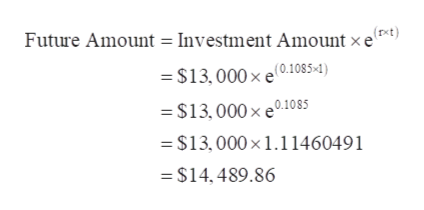# suppose you have\$13000 to invest. which of the two rates would yield the larger amount in one year: 11% compounded quarterly or 10.85% compounded continuously?

Question
2 views

suppose you have\$13000 to invest. which of the two rates would yield the larger amount in one year: 11% compounded quarterly or 10.85% compounded continuously?

check_circle

Step 1

Investment Amount (pv) = \$13,000

Interest Rate (rate) = 11%

Type of Interest Compounded = Quarterly

Time Period = 1 Year

Number of Payments in a Year = 4

Total Number of Payments (nper) = 1 × 4 = 4

Calculation of future amount of the investment using FV function of excel is as follows:

To open the "FV function" window - MS-Excel --> Formulas --> Financials --> FV.

Step 2

Investment Amount = \$13,000

Interest Rate (r) = 10.85% or 0.1085

Type of Interest Compounded = Continuously

Time Per...help_outlineImage TranscriptioncloseFuture Amount = Investment Amount x e (rxt) = \$13,000 × e(0.1085-4) = \$13,000 × e0.1085 = \$13,000×1.11460491 = \$14, 489.86 fullscreen

### Want to see the full answer?

See Solution

#### Want to see this answer and more?

Solutions are written by subject experts who are available 24/7. Questions are typically answered within 1 hour.*

See Solution
*Response times may vary by subject and question.
Tagged in